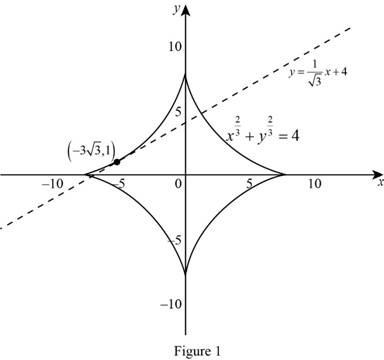# The equation of the tangent line to the given equation at the point.### Single Variable Calculus: Concepts...

4th Edition
James Stewart
Publisher: Cengage Learning
ISBN: 9781337687805### Single Variable Calculus: Concepts...

4th Edition
James Stewart
Publisher: Cengage Learning
ISBN: 9781337687805

#### Solutions

Chapter 3.5, Problem 26E
To determine

## To find: The equation of the tangent line to the given equation at the point.

Expert Solution

The equation of the tangent line to the equation x23+y23=4 at the point (33,1) is y=13x+4.

### Explanation of Solution

Given:

The curve is x23+y23=4.

The point is (33,1).

Derivative rules: Chain rule

If y=f(u) and u=g(x)  are both differentiable functions, then dydx=dydududx.

Formula used:

The equation of the tangent line at (x1,y1) is, yy1=m(xx1) (1)

Where, m is the slope of the tangent line at (x1,y1) and m=dydx|x=x1,y=y1.

Calculation:

Consider the equation x23+y23=4.

Differentiate the given equation implicitly with respect to x,

ddx(x23+y23)=ddx(4)ddx(x23)+ddx(y23)=023x231+ddx(y23)=023x13+ddx(y23)=0

Apply the chain rule and simplify the terms,

23x13+[ddy(y23)dydx]=023x13+[23y13dydx]=023x13+23y13dydx=023y13dydx=23x13

Separate dydx to one side of the equation,

23y13dydx=23x13dydx=23x1323y13dydx=x13y13dydx=y3x3

Therefore, the derivative of the equation is dydx=yx.

The slope of the tangent line at the point (33,1) is computed as follows,

dydx|(x,y)=(33,1)=13(33)13=1((1)333)13=1(333)13=13

Thus, the slope of the tangent line at (33,1) is m=13.

Substitute (33,1) for (x1,y1) and m=13 in equation (1),

y1=13(x+33)y1=13x+33(13)y1=13x+3y=13x+4

Therefore, the equation of the tangent line to the equation x23+y23=4 at the point (33,1) is y=13x+4.

Graph:

The graph of the curve and the tangent line is shown below in Figure 1.From Figure 1, it is observed that the line y=13x+4 is tangent to the curve x23+y23=4 at the point (33,1).

### Have a homework question?

Subscribe to bartleby learn! Ask subject matter experts 30 homework questions each month. Plus, you’ll have access to millions of step-by-step textbook answers!